# Lecture 2

## Compiling

• Last time, we learned to write our first program in C. We learned the syntax for the `main` function in our program, the `printf` function for printing to the terminal, how to create strings with double quotes, and how to include `stdio.h` for the `printf` function.
• Then, we compiled it with `clang hello.c` to be able to run `./a.out` (the default name), and then `clang -o hello hello.c` (passing in a command-line argument for the output’s name) to be able to run `./hello`.
• If we wanted to use CS50’s library, via `#include <cs50.h>`, for strings and the `get_string` function, we also have to add a flag: `clang -o hello hello.c -lcs50`. The `-l` flag links the `cs50` file, which is already installed in the CS50 Sandbox, and includes prototypes, or definitions of strings and `get_string` (among more) that our program can then refer to and use.
• We write our source code in C, but need to compile it to machine code, in binary, before our computers can run it.
• `clang` is the compiler, and `make` is a utility that helps us run `clang` without having to indicate all the options manually.
• “Compiling” source code into machine code is actually made up of smaller steps:
• preprocessing
• compiling
• assembling
• Preprocessing involves looking at lines that start with a `#`, like `#include`, before everything else. For example, `#include <cs50.h>` will tell `clang` to look for that header file first, since it contains content that we want to include in our program. Then, `clang` will essentially replace the contents of those header files into our program.
• For example …
``````#include <cs50.h>
#include <stdio.h>

int main(void)
{
string name = get_string("Name: ");
printf("hello, %s\n", name);
}
``````
• … will be preprocessed into:
``````string get_string(string prompt);
int printf(const char *format, ...);

int main(void)
{
string name = get_string("Name: ");
printf("hello, %s\n", name);
}
``````
• Compiling takes our source code, in C, and converts it to assembly code, which looks like this:
``````...
main:                         # @main
.cfi_startproc
# BB#0:
pushq    %rbp
.Ltmp0:
.cfi_def_cfa_offset 16
.Ltmp1:
.cfi_offset %rbp, -16
movq    %rsp, %rbp
.Ltmp2:
.cfi_def_cfa_register %rbp
subq    \$16, %rsp
xorl    %eax, %eax
movl    %eax, %edi
movabsq    \$.L.str, %rsi
movb    \$0, %al
callq    get_string
movabsq    \$.L.str.1, %rdi
movq    %rax, -8(%rbp)
movq    -8(%rbp), %rsi
movb    \$0, %al
callq    printf
...
``````
• These instructions are lower-level and is closer to the binary instructions that a computer’s CPU can directly understand. They generally operate on bytes themselves, as opposed to abstractions like variable names.
• The next step is to take the assembly code and translate it to instructions in binary by assembling it. The instructions in binary are called machine code, which a computer’s CPU can run directly.
• The last step is linking, where the contents of previously compiled libraries that we want to link, like `cs50.c`, are actually combined with the binary of our program. So we end up with one binary file, `a.out` or `hello`, that is the compiled version of `hello.c`, `cs50.c`, and `printf.c`.

## Debugging

• Bugs are mistakes in programs that we didn’t intend to make. And debugging is the process of finding and fixing bugs.

## help50 and printf

• Let’s say we wrote this program, `buggy0.c`:
``````int main(void)
{
printf("hello, world\n");
}
``````
• We see an error (in red), when we try to `make` this program, that we are `implicitly declaring library function 'printf'`. We don’t really understand this, so we can run `help50 make buggy0`, which will tell us, at the end, that we might have forgotten to write `#include <stdio.h>`, which contains `printf`.
• We can try this again with `buggy1.c`:
``````#include <stdio.h>

int main(void)
{
string name = get_string("What's your name?\n");
printf("hello, %s\n", name);
}
``````
• We see a lot of errors, and even the first one doesn’t seem to make much sense. So we can again run `help50 make buggy1`, which will hint to us that we need `cs50.h` since `string` isn’t defined.
• To clear the terminal window (so that we can see just the output of whatever we want to run next), we can press `control + L`, or type in `clear` as a command to the terminal window.
• Let’s look at `buggy2.c`:
``````#include <stdio.h>

int main(void)
{
for (int i = 0; i <= 10; i++)
{
printf("#\n");
}
}
``````
• Hmm, we intended to only see 10 `#`s, but there are 11. If we didn’t know what the problem is (since our program is compiling without any errors, and we now have a logical error), we could add another print line to help us:
``````#include <stdio.h>

int main(void)
{
for (int i = 0; i <= 10; i++)
{
printf("i is now %i: ", i);
printf("#\n");
}
}
``````
• Now, we see that `i` started at 0 and continued until it was 10, but we should have it stop once it’s at 10, with `i < 10` instead of `i <= 10`.

## debug50

• Today we’ll also take a look at CS50 IDE, which is like the CS50 Sandbox, but with more features. It is an online development environment, with a code editor and a terminal window, but also tools for debugging and collaborating: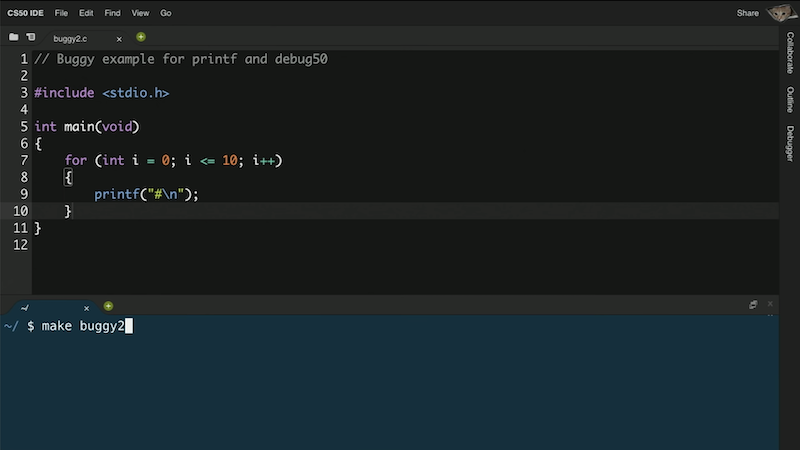• In the CS50 IDE, we’ll have another tool, `debug50`, to help us debug programs.
• We’ll open `buggy2.c` and try to `make buggy2`. But we saved `buggy2.c` into a folder called `src2`, so we need to run `cd src2` to change our directory to the right one. And CS50 IDE’s terminal will remind us what directory we’re in, with a prompt like `~/src/ \$`. (The `~` indicates the default, or home directory.)
• Instead of using `printf`, we can also debug our program interactively. We can add a breakpoint, or an indicator for a line of code where the debugger should pause our program. For example, we can click to the left of line 5 of our code, and a red circle will appear: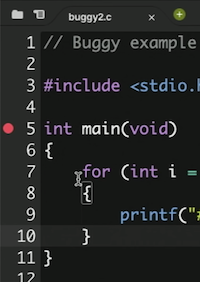• Now, if we run `debug50 ./buggy2`, we’ll see the debugger panel open on the right: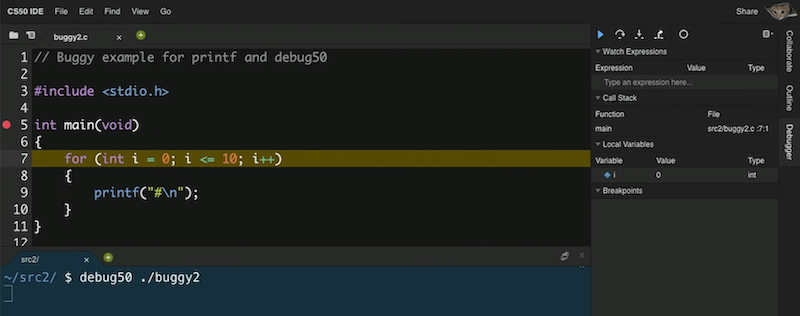• We see that the variable we made, `i`, is under the `Local Variables` section, and see that there’s a value of `0`.
• Our breakpoint has paused our program after line 5, to just before line 7, since it’s the first line of code that can run. To continue, we have a few controls in the debugger panel. The blue triangle will continue our program until we reach another breakpoint or the end of our program. The curved arrow to its right will “step over” the line, running it and pausing our program again immediately after.
• So, we’ll use the curved arrow to run the next line, and see what changes after. We’re at the `printf` line, and pressing the curved arrow again, we see a single `#` printed to our terminal window. With another click of the arrow, we see the value of `i` on the right change to `1`. And we can keep clicking the arrow to watch our program run, one line at a time.
• To exit the debugger, we can press `control + C` to stop the program.
• We can save lots of time in the future by investing a little bit now to learn how to use `debug50`!

## check50 and style50

• We can run a command like `check50 cs50/problems/hello`, where `check50` is a program that will follow instructions identified by the argument `cs50/problems/hello` to upload, run, and test our program on CS50’s servers. This will check our program for correctness.
• When writing software in the real world, developers will generally write their own tests to ensure their code works as they expect, especially as more features are added to the same code.
• `style50` is another program that will check our code for aesthetic issues, such as whitespace, such that our code is more readable and maintainable. For example, we might be missing indentation. And the Style Guide will include more explanations for what we expect.
• We can even use rubber duck debugging, a method where we explain what we’re trying to do to a rubber duck, such that we realize what we’re trying to do and what we should fix.
• We also want to write our code with good design, where we not only solve the problem correctly but well, where we make reasonable choices for how our program runs, and make tradeoffs between time, development cost, and memory.

## Data Types

• In C, we have different types of variables we can use for storing data:
• bool 1 byte
• char 1 byte
• int 4 bytes
• float 4 bytes
• long 8 bytes
• double 8 bytes
• string ? bytes
• Each of these types take up a certain number of bytes per variable we create, and the sizes above are what the sandbox, IDE, and most likely your computer uses for each type in C.

## Memory

• Inside our computers, we have chips called RAM, random-access memory, that stores data for short-term use. We might save a program or file to our hard drive (or SSD) for long-term storage, but when we open it, it gets copied to RAM first. Though RAM is much smaller, and temporary (until the power is turned off), it is much faster.
• We can think of bytes, stored in RAM, as though they were in a grid: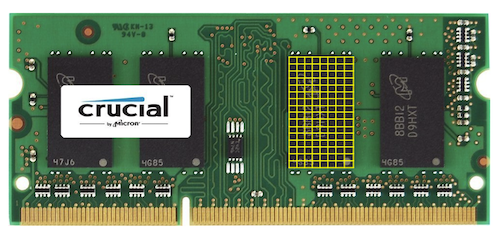• In reality, there are millions or billions of bytes per chip.
• In C, when we create a variable of type `char`, which will be sized one byte, it will physically be stored in one of those boxes in RAM. An integer, with 4 bytes, will take up four of those boxes.
• And each of these boxes is labeled with some number, or address, from 0, to 1, to 2, and so on.

## Arrays

• Let’s say we wanted to store three variables:
``````#include <stdio.h>

int main(void)
{
char c1 = 'H';
char c2 = 'I';
char c3 = '!';
printf("%c %c %c\n", c1, c2, c3);
}
``````
• Notice that we use single quotes to indicate a literal character, and double quotes for multiple characters together in a string.
• We can compile and run this, to see `H I !`.
• And we know characters are just numbers, so if we change our string formatting to be `printf("%i %i %i\n", c1, c2, c3);`, we can see the numeric values of each char printed: `72 73 33`.
• We can explicitly convert, or cast, each character to an int before we use it, with `(int) c1`, but our compiler can implicitly do that for us.
• And in memory, we might have three boxes, labeled `c1`, `c2`, and `c3` somehow, each of which representing a byte of binary with the values of each variable.
• Let’s look at `scores0.c`:
``````#include <cs50.h>
#include <stdio.h>

int main(void)
{
// Scores
int score1 = 72;
int score2 = 73;
int score3 = 33;

// Print average
printf("Average: %i\n", (score1 + score2 + score3) / 3);
}
``````
• We can print the average of three numbers, but now we need to make one variable for every score we want to include, and we can’t easily use them later.
• It turns out, in memory, we can store variables one after another, back-to-back. And in C, a list of variables stored, one after another in a contiguous chunk of memory, is called an array.
• For example, we can use `int scores;` to declare an array of 3 integers.
• And we can assign and use variables in an array with:
``````#include <cs50.h>
#include <stdio.h>

int main(void)
{
// Scores
int scores;
scores = 72;
scores = 73;
scores = 33;

// Print average
printf("Average: %i\n", (scores + scores + scores) / 3);
}
``````
• Notice that arrays are zero-indexed, meaning that the first element, or value, has index 0.
• And we repeated the value 3, representing the length of our array, in two different places. So we can use a constant, or fixed value, to indicate it should always be the same in both places:
``````#include <cs50.h>
#include <stdio.h>

const int N = 3;

int main(void)
{
// Scores
int scores[N];
scores = 72;
scores = 73;
scores = 33;

// Print average
printf("Average: %i\n", (scores + scores + scores) / N);
}
``````
• We can use the `const` keyword to tell the compiler that the value of `N` should never be changed by our program. And by convention, we’ll place our declaration of the variable outside of the `main` function and capitalize its name, which isn’t necessary for the compiler but shows other humans that this variable is a constant and makes it easy to see from the start.
• With an array, we can collect our scores in a loop, and access them later in a loop, too:
``````#include <cs50.h>
#include <stdio.h>

float average(int length, int array[]);

int main(void)
{
// Get number of scores
int n = get_int("Scores:  ");

// Get scores
int scores[n];
for (int i = 0; i < n; i++)
{
scores[i] = get_int("Score %i: ", i + 1);
}

// Print average
printf("Average: %.1f\n", average(n, scores));
}

float average(int length, int array[])
{
int sum = 0;
for (int i = 0; i < length; i++)
{
sum += array[i];
}
return (float) sum / (float) length;
}
``````
• First, we’ll ask the user for the number of scores they have, create an array with enough `int`s for the number of scores they have, and use a loop to collect all the scores.
• Then we’ll write a helper function, `average`, to return a `float`, or a decimal value. We’ll pass in the length and an array of `int`s (which could be any size), and use another loop inside our helper function to add up the values into a sum. We use `(float)` to cast both `sum` and `length` into floats, so the result we get from dividing the two is also a float.
• Finally, when we print the result we get, we use `%.1f` to show just one place after the decimal.
• In memory, our array is now stored like this, where each value takes up not one but four bytes: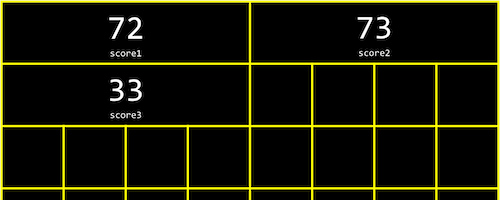## Strings

• Strings are actually just arrays of characters. If we had a string `s`, each character can be accessed with `s`, `s`, and so on.
• And it turns out that a string ends with a special character, ‘\0’, or a byte with all bits set to 0. This character is called the null character, or null terminating character. So we actually need four bytes to store our string “HI!”: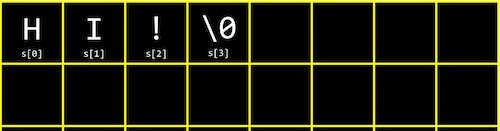• Now let’s see what four strings in an array might look like:
``````string names;
names = "EMMA";
names = "RODRIGO";
names = "BRIAN";
names = "DAVID";

printf("%s\n", names);
printf("%c%c%c%c\n", names, names, names, names);
``````
• We can print the first value in `names` as a string, or we can get the first string, and get each individual character in that string by using `[]` again. (We can think of it as `(names)`, though we don’t need the parentheses.)
• And though we know that the first name had four characters, `printf` probably used a loop to look at each character in the string, printing them one at a time until it reached the null character that marks the end of the string. And in fact, we can print `names` as an `int` with `%i`, and see a `0` being printed.
• We can visualize each character with its own label in memory: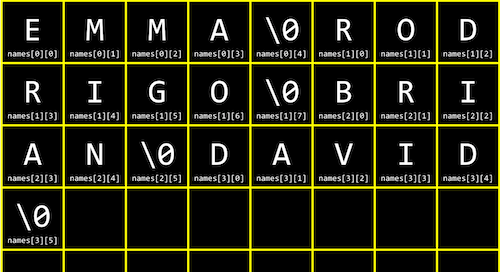• We can try experimenting with `string0.c`:
``````#include <cs50.h>
#include <stdio.h>
#include <string.h>

int main(void)
{
string s = get_string("Input:  ");
printf("Output: ");
for (int i = 0; i < strlen(s); i++)
{
printf("%c", s[i]);
}
printf("\n");
}
``````
• We can use the condition `s[i] != '\0'`, where we can check the current character and only print it if it’s not the null character.
• We can also use the length of the string, but first, we need a new library, `string.h`, for `strlen`, which tells us the length of a string.
• We can improve the design of our program. `string0` was a bit inefficient, since we check the length of the string, after each character is printed, in our condition. But since the length of the string doesn’t change, we can check the length of the string once:
``````#include <cs50.h>
#include <stdio.h>
#include <string.h>

int main(void)
{
string s = get_string("Input: ");
printf("Output:\n");
for (int i = 0, n = strlen(s); i < n; i++)
{
printf("%c\n", s[i]);
}
}
``````
• Now, at the start of our loop, we initialize both an `i` and `n` variable, and remember the length of our string in `n`. Then, we can check the values each time, without having to actually calculate the length of the string.
• And we did need to use a little more memory for `n`, but this saves us some time with not having to check the length of the string each time.
• We can now combine what we’ve seen, to write a program that can capitalize letters:
``````#include <cs50.h>
#include <stdio.h>
#include <string.h>

int main(void)
{
string s = get_string("Before: ");
printf("After:  ");
for (int i = 0, n = strlen(s); i < n; i++)
{
if (s[i] >= 'a' && s[i] <= 'z')
{
printf("%c", s[i] - 32);
}
else
{
printf("%c", s[i]);
}
}
printf("\n");
}
``````
• First, we get a string `s`. Then, for each character in the string, if it’s lowercase (its value is between that of `a` and `z`), we convert it to uppercase. Otherwise, we just print it.
• We can convert a lowercase letter to its uppercase equivalent, by subtracting the difference between their ASCII values. (We know that lowercase letters have a higher ASCII value than uppercase letters, and the difference is conveniently the same between the same letters, so we can subtract that difference to get an uppercase letter from a lowercase letter.)
• We can use the man pages, or programmer’s manual, to find library functions that we can use to accomplish the same thing:
``````#include <cs50.h>
#include <ctype.h>
#include <stdio.h>
#include <string.h>

int main(void)
{
string s = get_string("Before: ");
printf("After:  ");
for (int i = 0, n = strlen(s); i < n; i++)
{
printf("%c", toupper(s[i]));
}
printf("\n");
}
``````
• From searching the man pages, we see `toupper()` is a function, among others, from a library called `ctype`, that we can use.

## Command-line arguments

• We’ve used programs like `make` and `clang`, which take in extra words after their name in the command line. It turns out that programs of our own, can also take in command-line arguments.
• In `argv.c`, we change what our `main` function looks like:
``````#include <cs50.h>
#include <stdio.h>

int main(int argc, string argv[])
{
if (argc == 2)
{
printf("hello, %s\n", argv);
}
else
{
printf("hello, world\n");
}
}
``````
• `argc` and `argv` are two variables that our `main` function will now get, when our program is run from the command line. `argc` is the argument count, or number of arguments, and `argv` is an array of strings that are the arguments. And the first argument, `argv`, is the name of our program (the first word typed, like `./hello`). In this example, we check if we have two arguments, and print out the second one if so.
• For example, if we run `./argv David`, we’ll get `hello, David` printed, since we typed in `David` as the second word in our command.
• It turns out that we can indicate errors in our program by returning a value from our `main` function (as implied by the `int` before our `main` function). By default, our `main` function returns `0` to indicate nothing went wrong, but we can write a program to return a different value:
``````#include <cs50.h>
#include <stdio.h>

int main(int argc, string argv[])
{
if (argc != 2)
{
printf("missing command-line argument\n");
return 1;
}
printf("hello, %s\n", argv);
return 0;
}
``````
• The return value of `main` in our program is called an exit code.
• As we write more complex programs, error codes like this can help us determine what went wrong, even if it’s not visible or meaningful to the user

• A message like `HI!` could be converted to ASCII, `72 73 33`. But anyone would be able to convert that back to letters.
• For example, if we wanted to send a message like `I L O V E Y O U`, we can first convert it to ASCII: `73 76 79 86 69 89 79 85`. Then, we can encrypt it with a key of just `1` and a simple algorithm, where we just add the key to each value: `74 77 80 87 70 90 80 86`. Then, someone converting that ASCII back to text will see `J M P W F Z P V`. To decrypt this, someone will need to know the key.